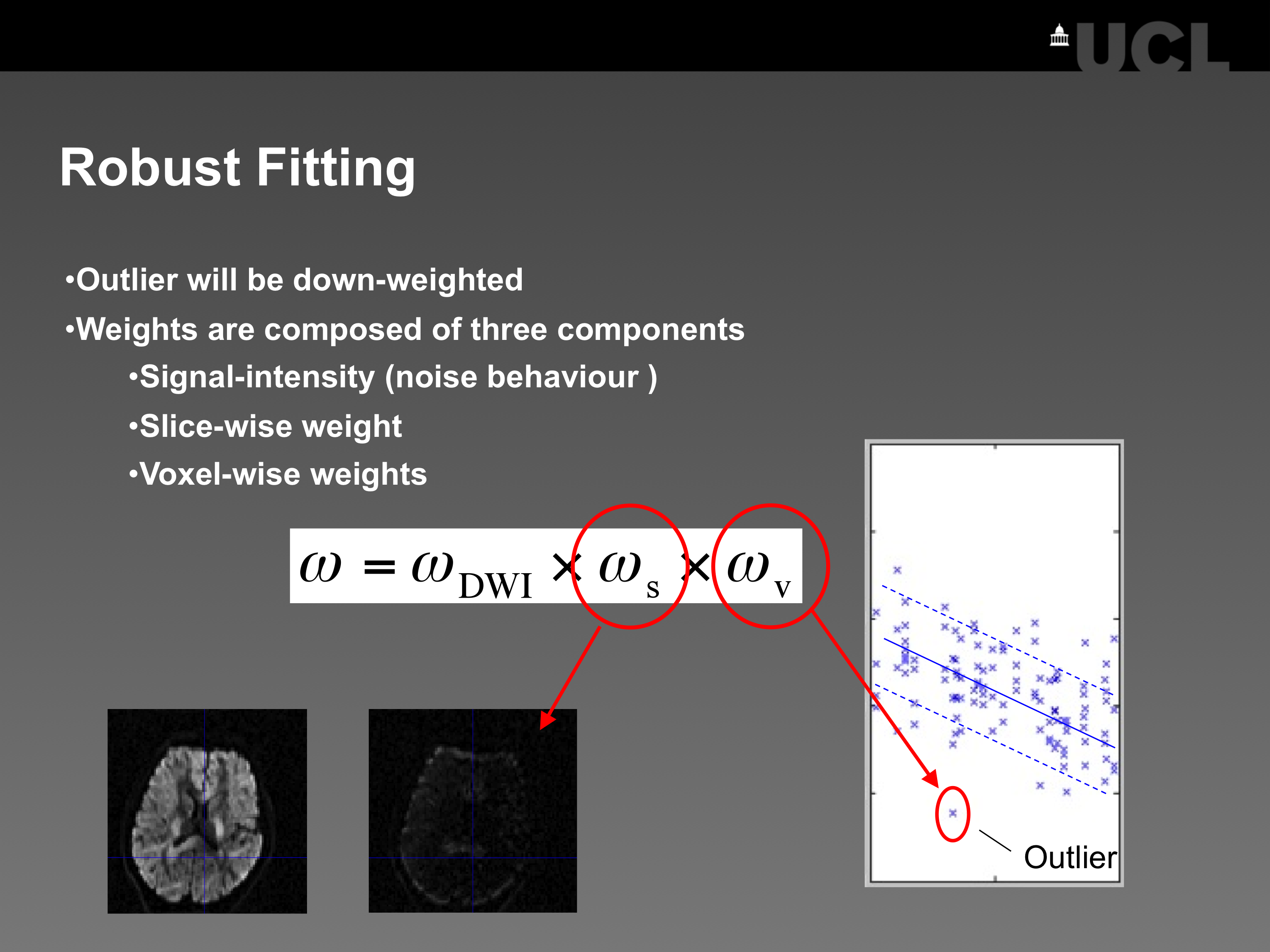## Tenor fitting

### What are the tensor fitting tools?

This tool uses the simplistic linear tensor approach to estimate the diffusion tensor and reject outliers. Currrently, the following methods are available to estimate the diffusion tensor: ordinary least squares, weighted least squares, and robust tensor fitting. Note that for data with low SNR (SNR < 4) the Gaussian noise distribution might be violated and the linear tensor fitting model might not be valid anymore.

### Use Tensor Fitting

Go to this wiki for instruction how to use this toolbox.

### A quick demo on how Robust Fitting worksFigure 1:

The robust tensor fitting method is a method that down-weights outliers in the diffusion signal. It is recommended if your data suffers from physiological noise or subject motion. It works well if the outliers are sparsely distributed along different diffusion directions (see, e.g. Zwiers (2010)) and you acquired enough data (N>30, where N is the number of images in the DTI sequence, see Chang et al. (2005)).

### Read more

Mohammadi S, Freund P, Feiweier T, Curt A, Weiskopf N, The impact of post-processing on spinal cord diffusion tensor imaging, NeuroImage 70: 377-385. doi:j.neuroimage.2012.12.058.